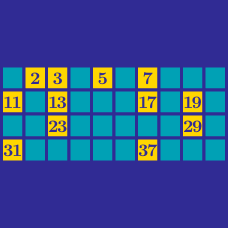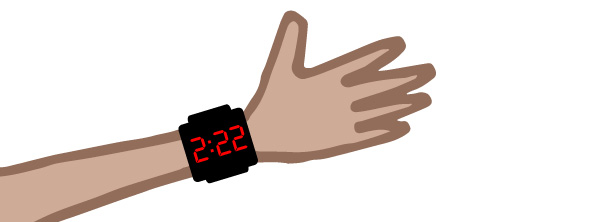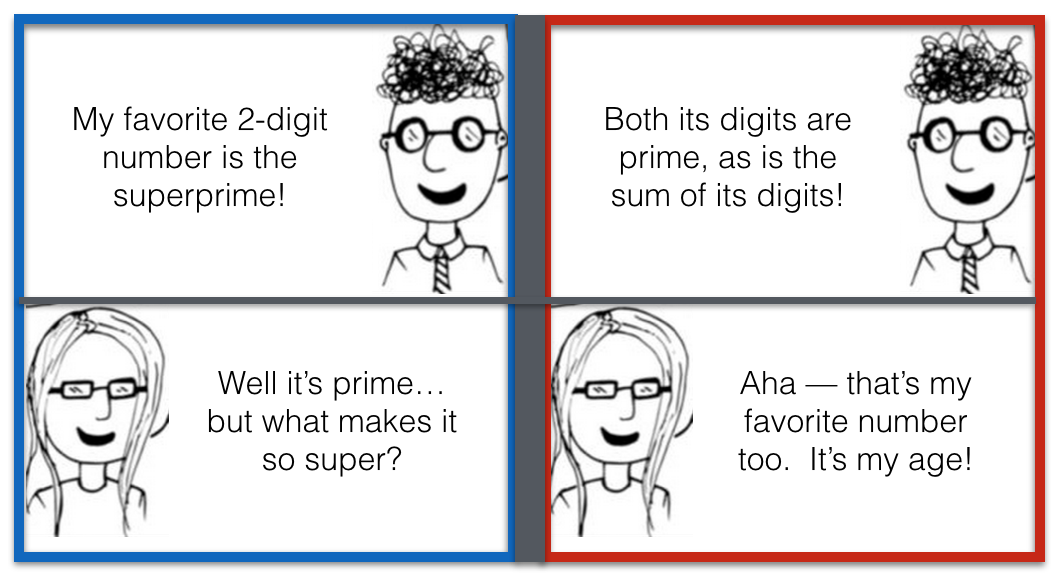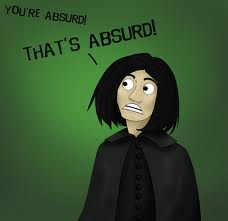Number Theory

# Prime Numbers: Level 1 ChallengesConsider a digital clock. The first time after midnight when the three digits are all prime numbers is 2:22 am. What is the last time before 10:00 am when the three digits on the clock are all prime numbers?

Details and assumptions

If your answer is 1:23 am, type it in as 123.John and Amy have the mathematical conversation above. How old is Amy?

The sum of three primes is 100. One of them exceeds the other by 36. Find the largest prime.

$345!+2 , \ \ 345!+3 , \ \ 345!+4,\ \ \ldots \ \ ,\ \ 345!+345$

Find the number of primes among the numbers above.If a prime $p$ can be expressed as the difference of square of two positive integers, then find the remainder, when $p^{2}+138$ is divided by $4$.

×